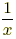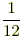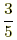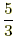# multiplicative inverse of a number

Multiplicative Inverse of a Number
Reciprocal

The reciprocal of x is. In other words, a reciprocal is a fraction flipped upside down. Multiplicative inverse means the same thing as reciprocal.

For example, the multiplicative inverse (reciprocal) of 12 isand the multiplicative inverse (reciprocal) ofis.

Note: The product of a number and its multiplicative inverse is 1. Observe that·= 1.